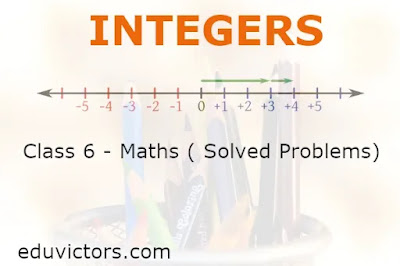# Class 6 Maths - Integers ( Questions and Answers)

Important Points:

The collection of numbers ....−2, −1, 0, 1, 2 .... is called integers.

The numbers with the negative sign are called negative integers and the numbers with positive sign are called positive integers.

One more than the given number gives successor and one less than the given number gives predecessor.

We can do addition and subtraction of integers on a number line.

Additive inverse: It is the number which when added to any integer gives the result 0.

To subtract an integer from another integer it is enough to add the additive inverse of the integer that is being subtracted, to the other integer.

Q1: Write opposites of the following:

(a) Increase in weight

(b) 30 km north

(c) 100 m above sea level

(d) Loss of Rs 700

(a) Decrease in weight

(b) 30 km south

(c) 100 m below sea level

(d) Gain of Rs 700

Q2: Represent the following numbers as integers with appropriate signs.

(a) An aeroplane is flying at a height two thousand metre above the ground.

(b) A submarine is moving at a depth, eight hundred metre below the sea level.

(c) A deposit of rupees two hundred.

(d) Withdrawal of rupees seven hundred.

(e) ₹ 45 profit is represented by +45 then ₹ 36 loss is represented by ______.

(a) +2000

(b) −800

(c) +200

(d) −700

(e) -36

Q3: Represent the following numbers on a number line:

(a) + 5 (b) -10

Q4: Adjacent figure is a vertical number line, representing integers. Observe it and locate the following points:

(a) If point D is + 8, then which point is − 8?

(b) Is point G a negative integer or a positive integer?

(c) Write integers for points B and E.

(d) Which point marked on this number line has the least value? (e) Arrange all the points in decreasing order of value.

(a) F

(b) Negative integer (−6)

(c) Point B represents 4 and point E represents −10.

(d) E has the least value as it represents −10.

(e) D > C > B > A > O > H > G > F > E

Q5: In each of the following pairs, which number is to the right of the other on the number line?

(a) 2, 9

(b) − 3, − 8

(c) 0, − 1

(a) As,9>2

So, 9 is to the right side of 2 on number line.

(b) As, −3 > −8

So, −3 is to the right side of −8 on number line.

(c) As,0>−1

So, 0 is to the right side of −3 on number line.

Q6: Arrange the following in ascending order: –3, –8, 10, –2, 7, 15, –12, 6.

Answer: –12, –8, –3, –2, 6, 7, 10, 15.

Q7: Write all the integers between the given pairs (write them in the increasing order.)

(a) 0 and − 7

(b) − 4 and 4

(c) − 8 and − 15

(a) −6 < −5 < −4 < −3 < −2 < −1

(b) −3 <−2 < −1 < 0 < 1 < 2 < 3

(c) −14 < −13 < −12 < −11 < −10 < −9

Q8: With the help of a number line answer the following:

(a) Which number will we reach if we move 4 numbers to the right of − 2.

(b) Which number will we reach if we move 5 numbers to the left of 1.

(c) If we are at − 8 on the number line, in which direction should we move to reach − 13?

(d) If we are at − 6 on the number line, in which direction should we move to reach − 1?

(a) We will reach at 2, if we move 4 numbers to the right of −2.

(b) We will reach at −4, if we move 5 numbers to the left of 1.

(c) Clearly, −13 is to the left of −8. Therefore, we should move towards the left direction.

(d) Clearly, −1 is to the right of −6. Therefore, we should move towards the right direction

Q9: (a) Write four negative integers greater than − 20.

(b) Write four negative integers less than − 10.

(a) −19, −18, −17, −16

(b) −11, −12, −13, −14

Q10(MCQ): Write the correct answer from the given four options:

Sania and Trapi visited Leh and Tawang respectively during winter. Sania reported that she had experienced –4°C on Sunday, while Trapi reported that she had experienced –2°C on that day. On that Sunday

(A) Leh was cooler than Tawang.

(B) Leh was hotter than Tawang.

(C) Leh was as cool as Tawang.

(D) Tawang was cooler than Leh.

Q11: State whether each of the following statements is true or false:

(a) Every positive integer is greater than 0.

(b) Every integer is either positive or negative.

(a) True

(b) False

Q12(MCQ): Every integer less than 0 has the sign

(A) +

(B) –

(C) ×

(D) ÷

Q13: Is zero positive or negative?

Answer: Zero corresponds to the origin. This is the reference point. This does not involve any direction. Therefore zero is neither positive nor negative.

Q14: Kamal deposited Rs. 5000 in the savings account. He withdrew Rs. 1500. Use positive and negative numbers for the above statement.

Kamal deposited : + Rs. 5000

Kamal withdrew : – Rs. 1500